### Reverse-engineering an early calculator chip with four-phase logic

In 1969, high-density MOS integrated circuits were still new and logic circuits were constructed in a variety of ways. One technique was "four-phase logic", which provided ten times the speed and density of standard logic gates while using 1/10 the power.1 One notable application of four-phase logic was calculators. In 1969, Sharp introduced the first calculator built from high-density MOS chips, the QT-8D, followed by the world's smallest calculator, the handheld EL-8. These calculators were high-end products, selling for \$345 (about \$1800 today).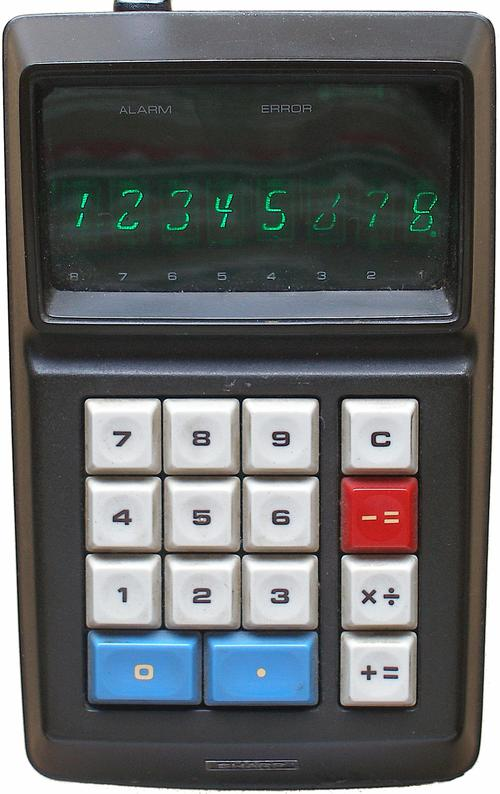The Sharp EL-8 calculator. Note the unusual 8-segment display for the digits. Photo by Felix Maschek, CC BY-SA 3.0 DE.

Integrated circuits at the time weren't dense enough to implement an entire calculator on one chip so these calculators split the functionality across five ICs. These five chips were created for Sharp by the Autonetics division of Rockwell. Autonetics invented four-phase logic in the mid-1960s, so this logic family was a natural choice for the calculator chips.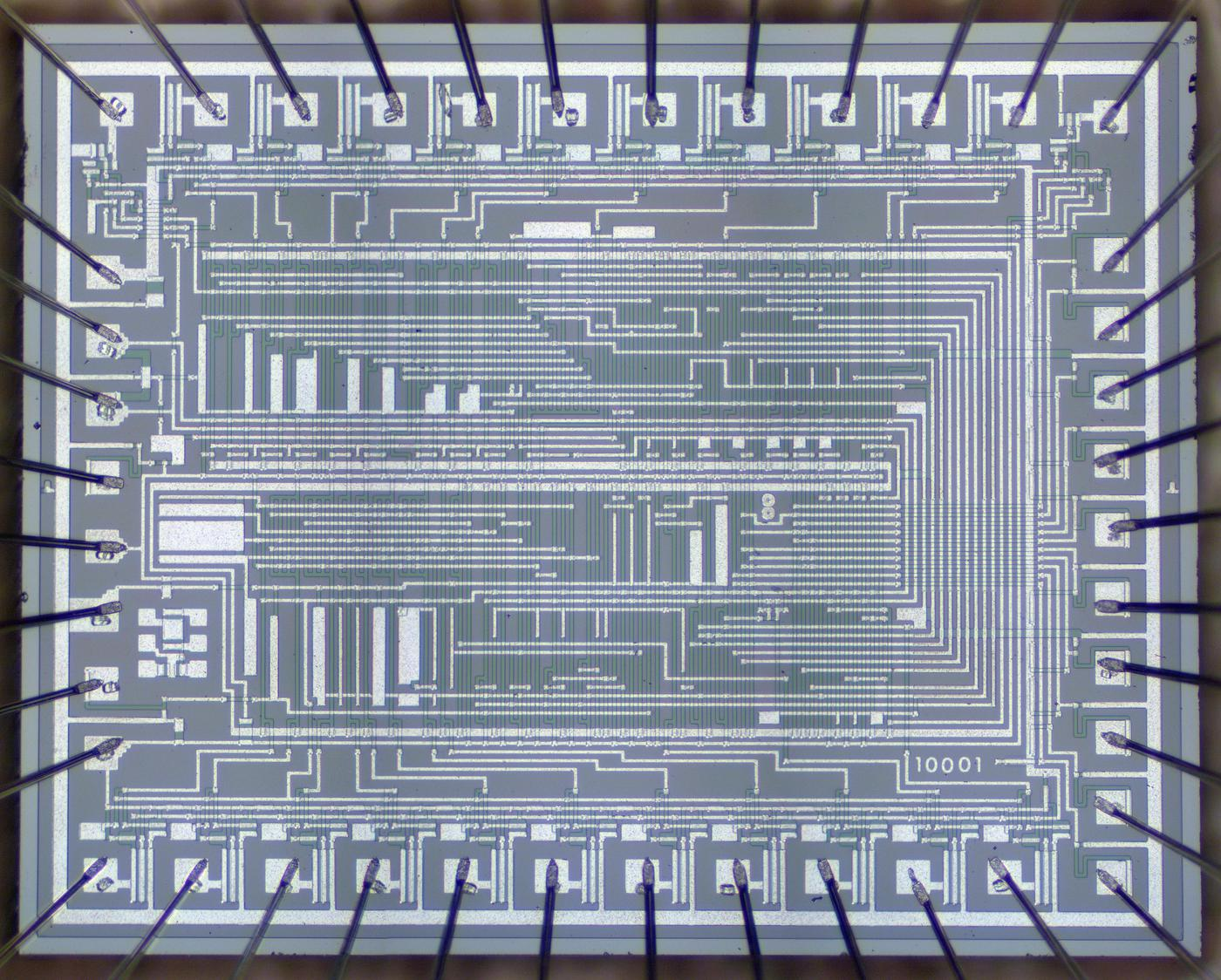Die photo of the NRD2256 keypad/display chip. Die photos courtesy of François Gueissaz.

In this blog post, I reverse-engineer the keypad/display chip shown above. This photo shows the tiny silicon die under a microscope. The silicon substrate has a purple tint while the doped, conductive silicon is green. The metal layer on top is white. Around the edges, thin bond wires connect the die to the 42 external pins. The chip contains roughly 500 transistors implementing 100 logic gates. While the density of this chip is absurdly low by modern standards, it illustrates the progress of MOS integrated circuits in the late 1960s.

## Inside the calculator

The photo below shows the circuit board inside the calculator. The board is dominated by the four large integrated circuits with circular golden lids. These integrated circuits were packaged as 42-pin ceramic ICs with staggered pins, an arrangement that provided more room for the PCB traces. Unlike modern printed circuit boards, the traces on this board are curved, showing its hand-drawn layout.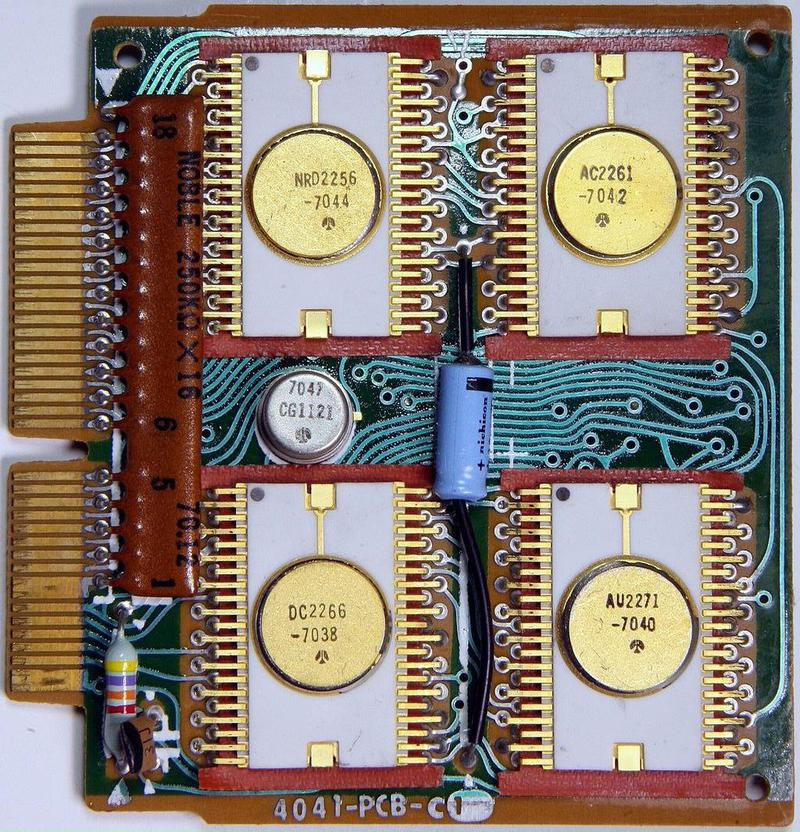The circuit board for the Sharp EL-8 calculator. The clock IC is the small metal-can package in the middle. Photo from Mister rf (CC BY-SA 4.0).

These four chips have different functions: an arithmetic chip, a decimal point chip, a keypad/display chip, and a control chip. This blog post focuses on the keypad/display chip (NRD2256) in the upper left. The fifth chip, is the clock chip in the small metal can that provides the four-phase timing pulses. The system clock runs at about 60 kilohertz, very slow by microprocessor standards, but fast enough for a calculator

One function of the keypad/display chip is to handle keypresses, converting a digit key into a 4-bit serial binary value. (Unexpectedly, non-digit keypresses are handled by other chips.) Its second main function is to display digits on the display. Like most calculators, this calculator multiplexes the display; it displays one digit at a time, repeated rapidly enough that the display appears uniform. It does this by activating one display tube at a time and energizing the appropriate segments to produce the desired digit.2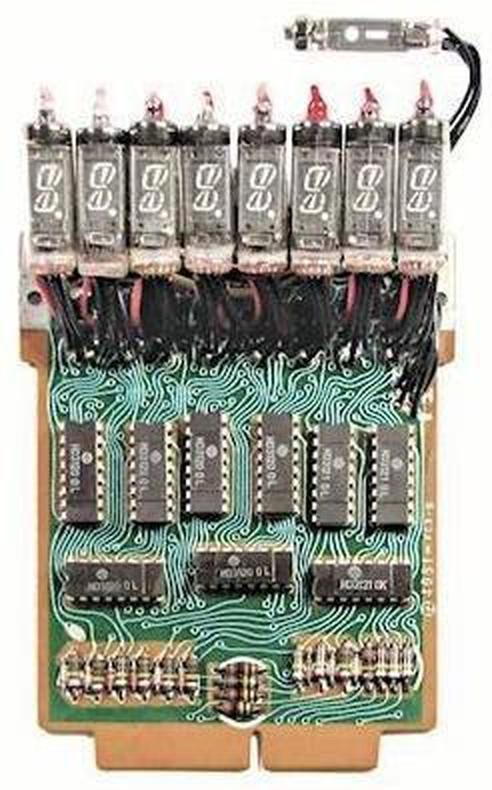Display board for the Sharp EL-8 calculator. Note the special tube at the top that displays a minus sign and error dot. Photo courtesy of John Wolff's Web Museum © 2012.

The four main chips communicate serially, sending each decimal digit as four BCD (binary-coded decimal) bits. Each communication cycle consists of 8 digits plus a ninth unused spot, forming a 36-bit "packet".3 The basic timing comes from the 60-kilohertz clock chip; one bit is sent each clock cycle. The keypad/display chip produces additional timing signals keep everything synchronized. First, it divides the clock by 4, generating a "digit clock" signal that indicates each 4-bit digit. The keypad/display chip cycles through the display digits, one digit every four clocks; it transmits signals to the other chips to keep track of the current digit. Thus, as the keypad/display chip cycles through the digits of the display, it receives the binary value of each digit at the right time.

The diagram below shows the functional units in the keypad/display chip. The "digit scan" circuitry scans through the eight digit drive lines D1-D8. The "decimal point" circuitry deserializes the decimal point input "dp" and energizes the decimal point segment when the specified digit is active. The "digit serialize" circuit converts a digit keypress into four serial bits. The "wiring" section is simply wiring between the upper half of the chip and the lower half, showing how much space is wasted by signal routing. In the lower half, the "9-segment decoder" illuminates the appropriate segments to display a digit; this digit is serialized by the "digit latch" circuit. The "clk÷4" circuit divides the input clock by four to produce the digit clock. Finally, the "key encode" circuit converts a keypress (0-9) into the four-bit value used by the "digit serialize" circuit. As will be seen, these functional blocks are not very complex, consisting of maybe 20 gates each.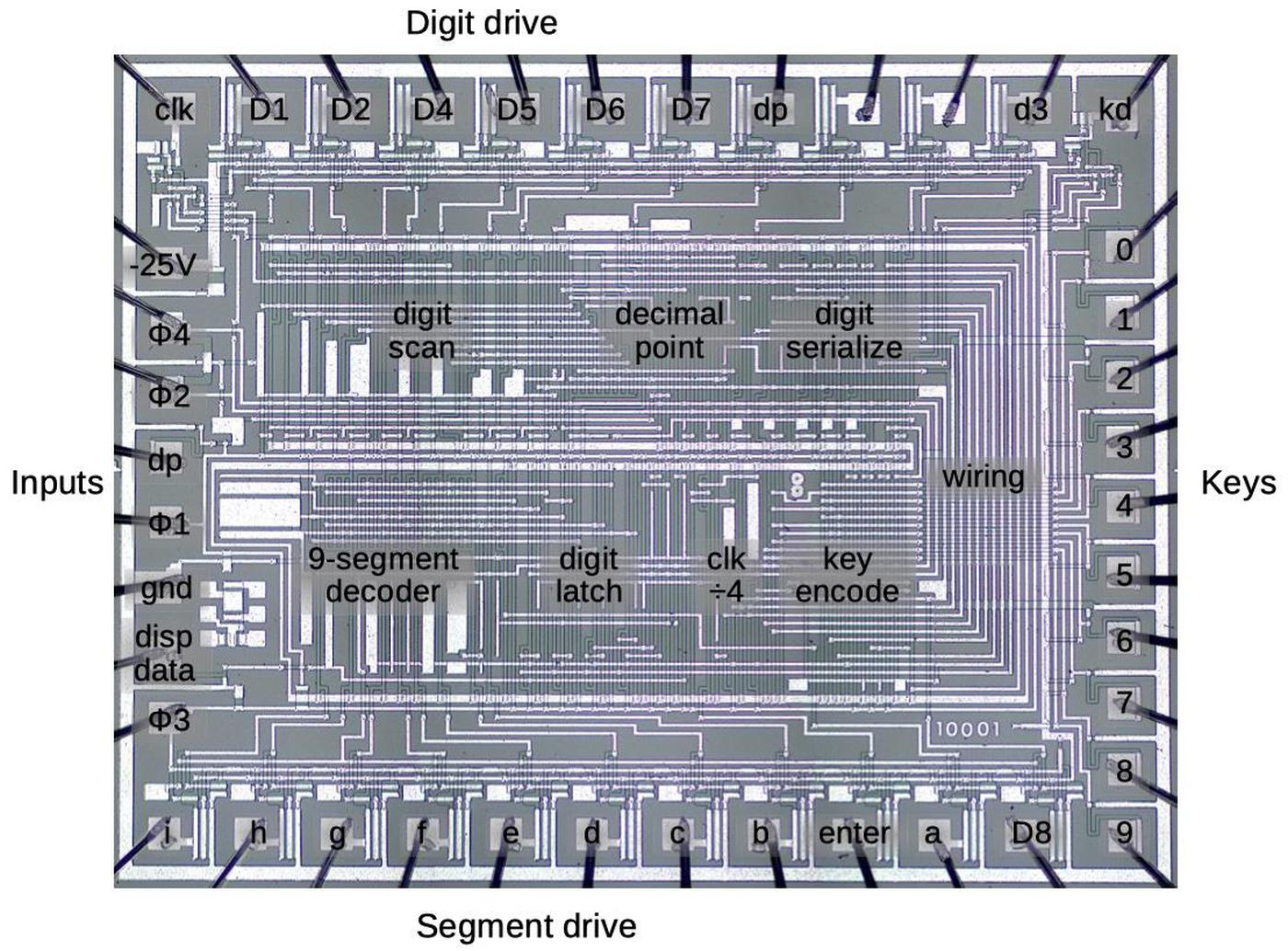The die of the NRD2256 keypad/display chip with the functional blocks labeled.

## PMOS Transistors

The calculator chip is built from metal-gate PMOS transistors. This type of transistor was easy to manufacture in the 1960s, but rapidly became obsolete. These transistors required large negative voltages, -25 volts for the calculator chip. (For simplicity, I will view the signals as active-low; 0V is a logical 0 and -25V is a logical 1.) Another problem with metal-gate transistors is that most of the chip was occupied by silicon and metal wiring, so the density of transistors was very low.

The diagram below illustrates a metal-gate PMOS transistor. At the bottom, two regions of silicon (green) are doped to make them conductive, forming the source and drain of the transistor The gate is formed by a metal strip between the silicon regions, separated from the silicon by a thin layer of insulating oxide. (These layers—Metal, Oxide, Semiconductor—give the MOS transistor its name.) The transistor can be considered a switch between the source and drain, controlled by the gate. To simplify the behavior, a PMOS transistor turns on when the gate is pulled negative (-25 volts), while the transistor turns off when the gate is at 0 volts.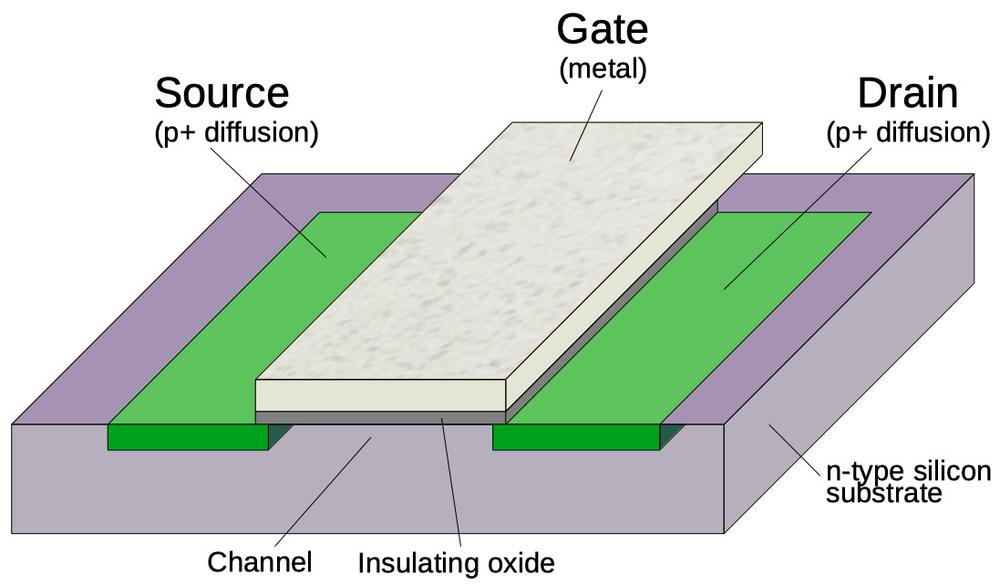Structure of a PMOS metal-gate transistor.

The image below shows how a transistor appears on the die. The gate is formed by the metal overlapping the doped silicon (vertical green strip). Inconveniently, a contact that connects the metal layer to the silicon looks very similar to a transistor in this chip—the metal layer in a transistor almost touches the silicon, while the metal layer in a contact touches the silicon. A contact and a transistor can be distinguished with effort; a contact is more square-shaped while a transistor is more oval-shaped and slightly blurrier. As will be explained below, four-phase logic often uses transistors where both the gate and the drain are connected to the same clock; this type of connection appears at the bottom of the diagram. By recognizing the transistors, the circuitry can be reverse-engineered.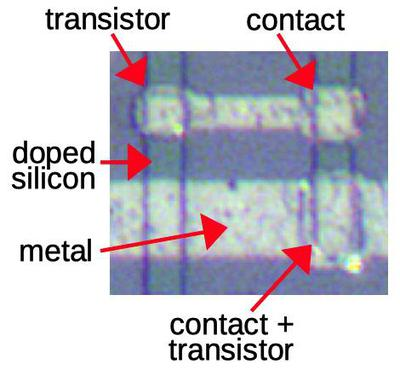Transistors look similar to metal/silicon contacts, but have subtle differences.

## How four-phase logic works

Four-phase logic is a technique for building logic gates, such as NAND gates. At the time, the standard way of building a logic gate was called "static logic", because the output remained constant as long as the inputs didn't change. A disadvantage of static logic was that it required a large "load transistor" that continuously used current, resulting in high power consumption.

A solution to these problems was "dynamic logic". Instead of providing a steady output from the gate, the gate's output was controlled by a clock signal. The gate's value would be computed and then stored by the circuit's capacitance, instead of requiring a continuous current. Developing with dynamic logic can be tricky, however, because of its dependence on timing. (It also has the disadvantage that the output values rapidly leak away, rather than being stable as with static logic.) Dynamic logic is still used in modern CPUs, in the form of domino logic.

Four-phase logic is a specific type of dynamic logic, designed to simplify the design process. Its timing is controlled by four clock signals (below), the source of the name "four-phase".4 In the calculator, these clock signals repeated at 60 kilohertz.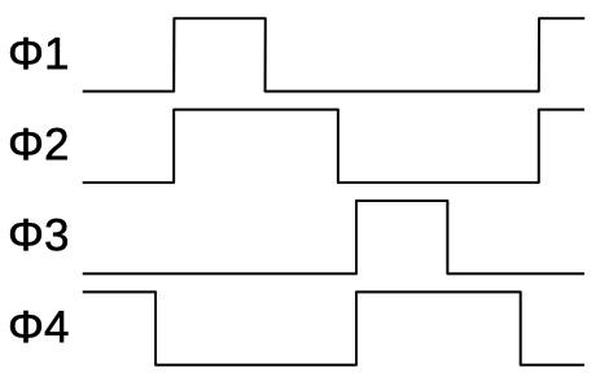This shows one cycle of the four-phase clock. The four-phase clock consists of four clock signals in this specific pattern.

The diagram below shows how an inverter is implemented in four-phase logic. In the first clock phase, φ1 is high causing the capacitor to get charged. In the second clock phase, the gate's value is determined. If the input is 0, the capacitor keeps its previous value (1). But if the input is 1, the capacitor discharges through the lower transistors so the output is 0. Thus, the circuit inverts the input.5 The capacitor holds the output for the remainder of the clock cycle, so the gate also acts as a latch. (This is an important feature of four-phase logic, simplifying many circuits.)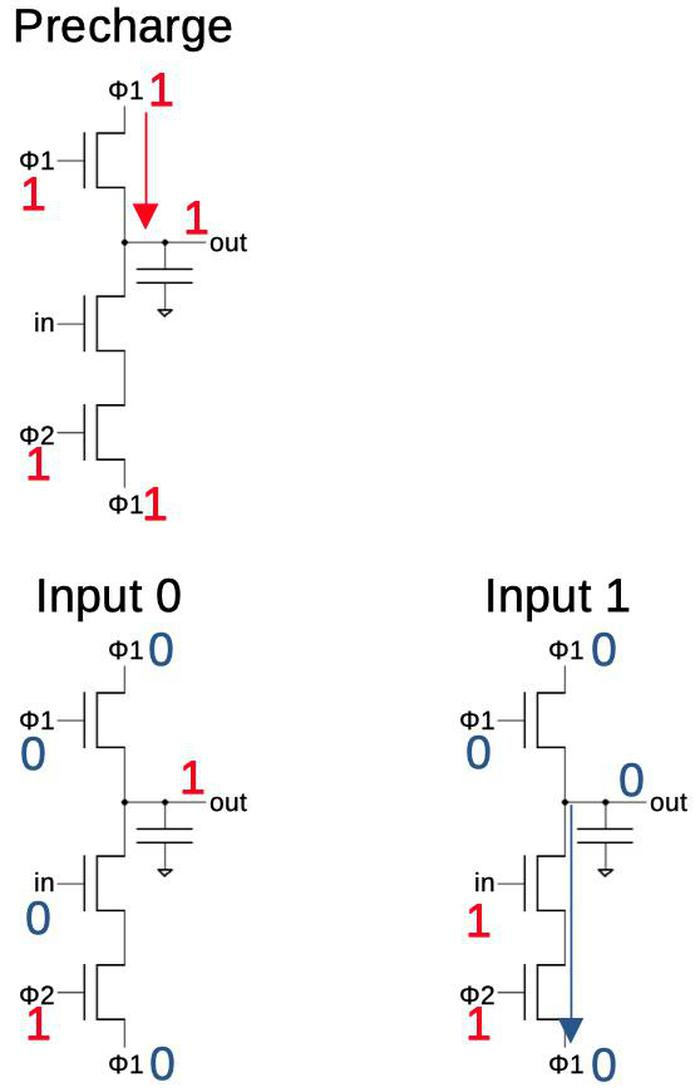Operation of a four-phase inverter. The gate is first precharged. In the evaluation step, the gate either remains charged (if the input is 0) or is discharged (if the input is 1).

More complex gates are built in a similar manner. For a NAND gate, multiple input transistors are put in series; if all inputs are 1, the capacitor will discharge and the output will be 0. For a NOR gate, multiple input transistors are put in parallel; any 1 input will yield a 0. As will be seen later, complex gates can be created with a mixture of series and parallel transistors.

The gate described above only uses two phases,6 so why four-phase logic? The problem with the above circuit is that if you connect two gates together, during step 2 the output of the first gate will be changing while the second gate is using this value. This could cause the second gate to erroneously discharge, yielding the wrong answer. The solution is for the second gate to wait until the first gate is stable. Specifically, the first gate operates during time periods 1 and 2, while the second gate operates during time periods 3 and 4. The second gate can then be safely connected to another gate operating during time periods 1 and 2. A circuit that alternates the two types of gates will operate safely.7

The diagram below shows how a four-phase inverter appears on the die. The schematic is the same as before, but the circuit is stretched vertically, with a layout that is tall and skinny. The inverter consists of a doped silicon line (green) running vertically, crossed by metal wiring. The gate is implemented by three small transistors. The large capacitor in the middle holds the output voltage. Dynamic logic is often built to use the stray capacitance of the wiring, but this chip uses many large capacitors (perhaps due to leakage or the slow clock speed).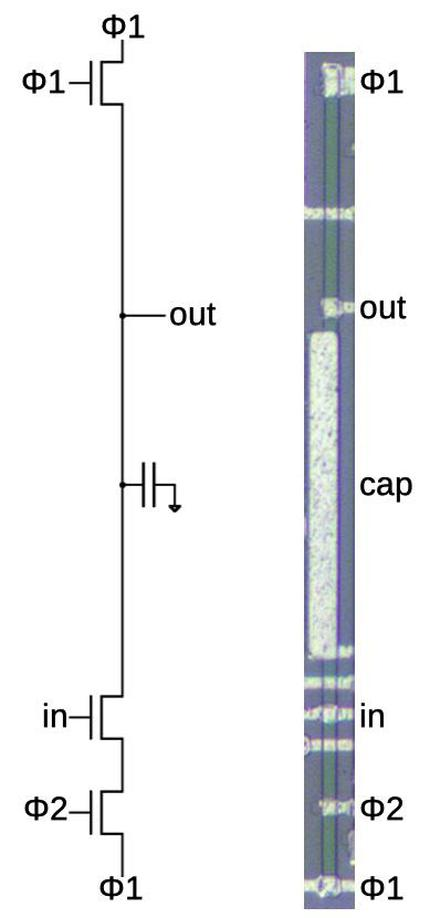An inverter on the die of the calculator chip.

## Implementation of the calculator circuits

In the next sections, I'll describe how some of the calculator IC's circuits are implemented using four-phase logic.

### Shift register

This chip uses shift registers to convert a serial input signal into a parallel binary value. One shift register is used for the decimal point position input while another shift register handles the digit to be displayed. The basic implementation of the shift register is a chain of inverters with two inverters per stage. Because four-phase logic is clocked, a bit will advance through the two inverters every clock cycle. (One inverter during Φ1/Φ2 and the second inverter during Φ3/Φ4.) This is an advantage of four-phase logic; standard logic requires a flip-flop at each stage to hold the bits, making the circuit much more complex. Each stage has an additional inverter to output the uncomplemented value. To keep both outputs synchronized, these inverters use special timing, precharging on Φ3 and reading on Φ1.7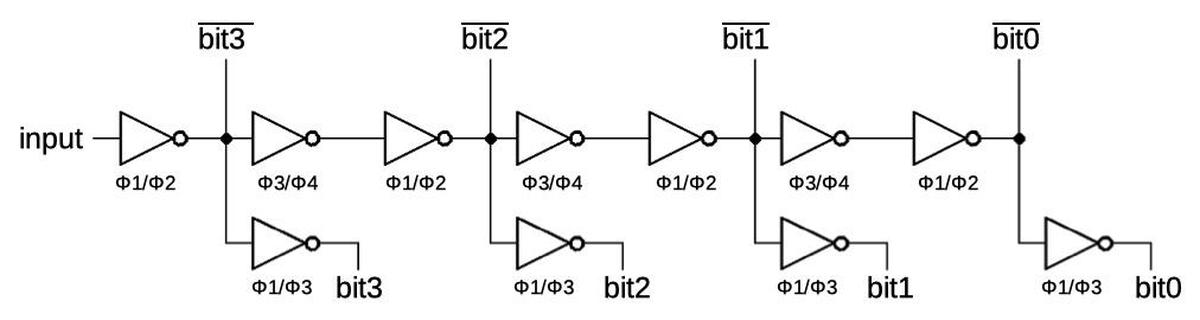A shift register, built from inverters.

The diagram below shows how the shift register for the decimal point position is implemented on the die. It shows nine inverters, implemented with 27 transistors. Each vertical green line of doped silicon is one gate, while the white metal wiring is mostly horizontal. Note that this circuitry, just nine gates, takes up a large fraction of the die. While the gates are tightly packed side-to-side, they are very tall, so the die holds just two rows of gates. The density of transistors is very low, with most of the area consumed by wiring. Even so, four-phase logic was considered a dense way of creating gates, since other techniques were even worse. (A couple of years later, microprocessors used an additional layer of polysilicon wiring, which made signal routing much easier and greatly increased the density.)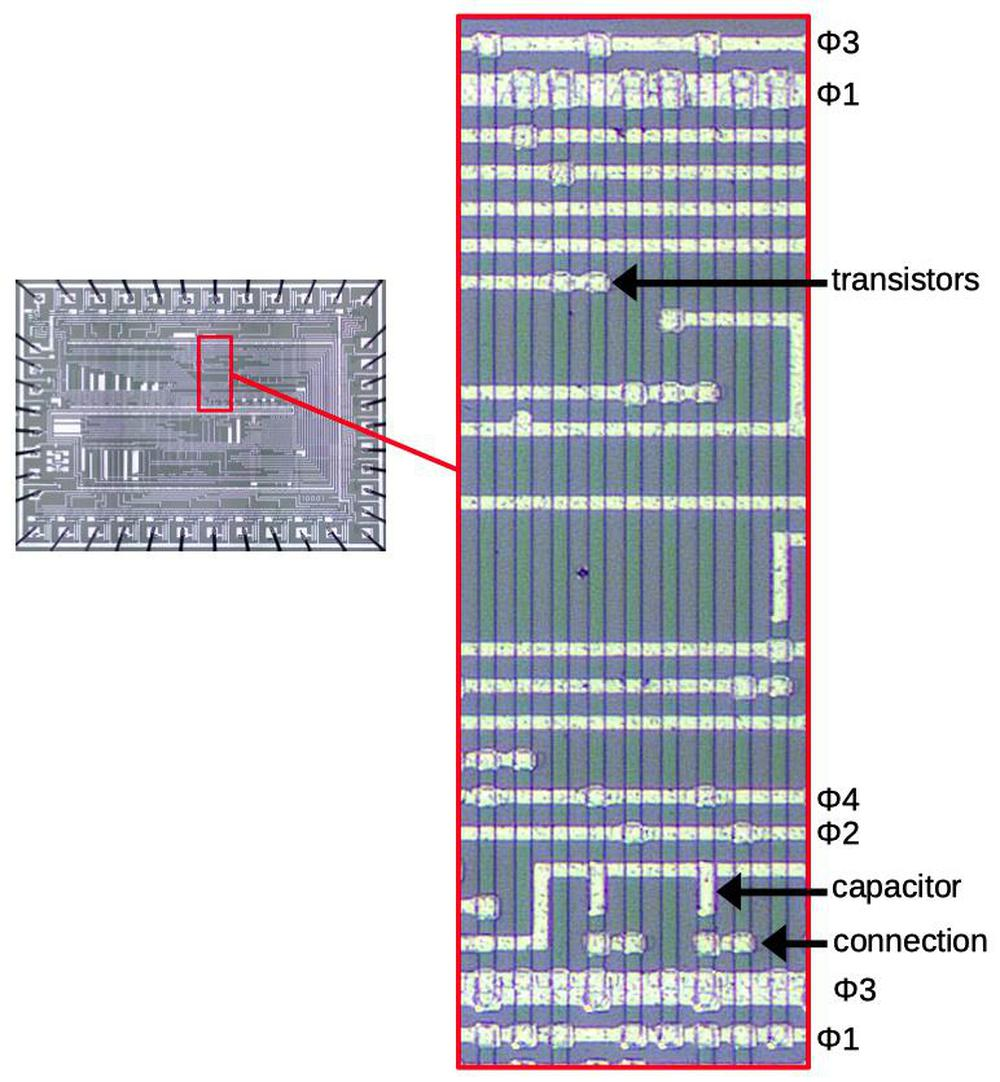A shift register as implemented on the die.

Examples of transistors and capacitors are indicated on the diagram. At the bottom, the arrow shows one of the connections between two inverters. The short horizontal wire is connected to the inverter on the left, and forms the gate of the inverter on the right. Other wires are longer as they connect inverters to other parts of the circuitry.

### Binary encoding

The chip converts each digit keypress into a binary encoding, using the NAND gates shown below. The calculator's buttons are magnets, closing reed switches. These switches are connected to the inputs on the right. When a key is pressed, the input goes low and the circuit generates the corresponding 4-bit binary output at the bottom.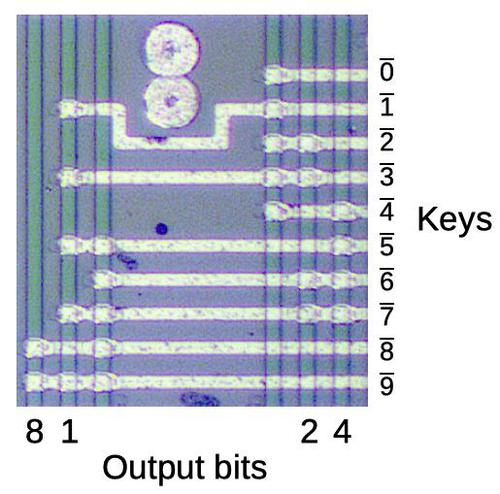The key encoder uses NAND gates to convert key presses into the binary encoding. The circles are probably mask alignment marks.

Each vertical green line corresponds to a NAND gate. (These gates are tall like the previous ones, but I'm only showing the interesting part.) The interesting thing about the encoder is that the binary representation is visible in the transistor pattern. For instance, the "1" bit output is connected to alternating inputs, while the "4" bit output is activated by keys 4 through 7. The unlabeled lines are used to determine if any key is pressed.

### Segment decoder

The desktop QT-8D calculator uses an unusual 9-segment display with curved segments, while the handheld EL-8 used an 8-segment display (omitting segment i, which provided a tail on the 4). These produce curved digits, unlike the blocky 7-segment digits seen in most calculators. The zero is particularly unusual: it is half-height. The calculator doesn't suppress leading zeroes, so the half-height zeros are less obtrusive. (1234, for instance, appears as oooo1234.)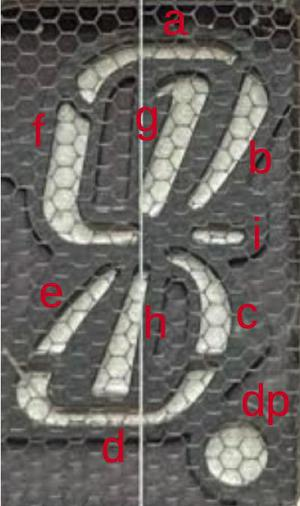The 9-segment vacuum fluorescent display tube used in the QT-8D calculator. The vertical line down the middle is the heated cathode and the hex mesh is the grid.

The role of the segment decoder is to take a binary value and drive the appropriate segments, labeled a through i. The circuit below is the interesting part of the decoder circuit. The bit values and their complements enter on the right from the shift register. Most of the segments are decoded by AND-NOR gates; an AND-NOR gate consists of several AND terms with the results NOR'd together. An AND-NOR gate is implemented in four-phase logic as a single gate with a separate vertical strip for each AND term. The strips are tied together at the top and bottom so if any strip is activated, the gate is discharged; this provides the NOR action. As a result, the physical structure of the gate maps directly to its logical structure.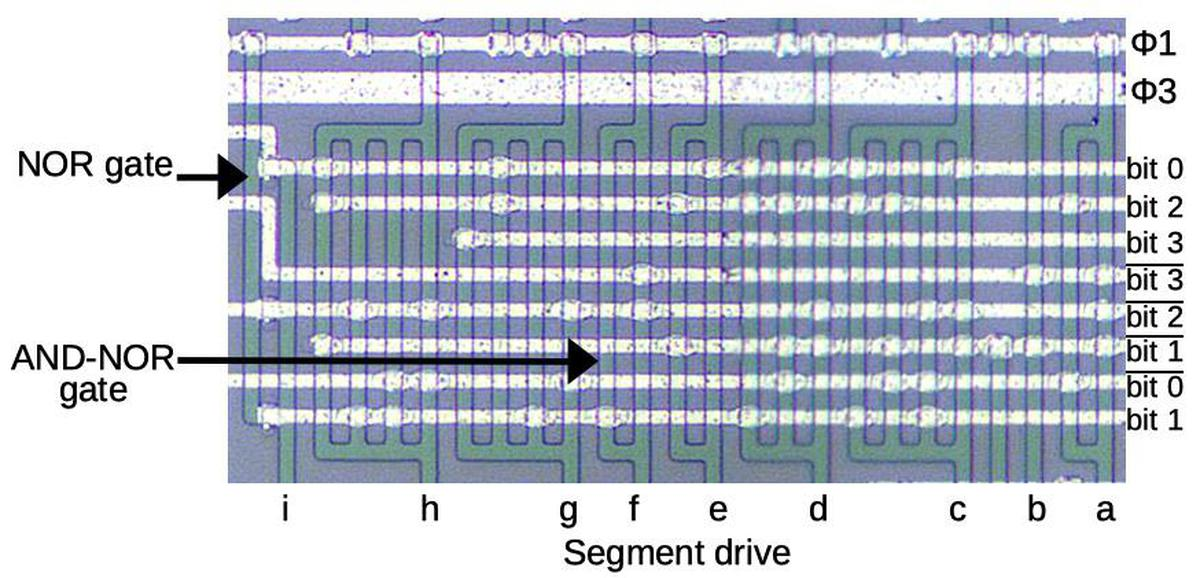Part of the segment decoder circuitry.

The gate for segment f is indicated on the diagram by an arrow. It has two vertical strips, so two AND terms. Studying the transistor connections, this gate implements: bit1 NOR (bit3 AND bit2). Evaluating this expression shows that f will be active for the digits 4, 5, 8, and 9. Looking at the display, you can verify that these are the digits that use segment f. Similar expressions are used to generate the other segments. For instance, segment h has four AND terms.

Segment i is activated by a NOR gate, which has two parallel vertical segments with three transistors in between. If any transistor is activated, it will connect the segments and discharge the gate, providing the NOR action. NOR gates are rare on the chip, probably because they require twice the width of a NAND gate. Segment i is NOR(bit0, bit2, bit1), so it is activated only for the number 4; this segment provides a short tail on the displayed 4.

### Decimal point decoding

One of the tasks of this chip is to display the decimal point, which is more complex than you might expect. The decimal point is encoded as a 4-bit value, transmitted serially to the chip. Three bits indicate the position of the decimal point (0 to 7), while the fourth bit enables or disables the decimal point. A shift register (described earlier) converts the serial bits to a 4-bit value. A remarkably complex gate (below) is used to determine when the active digit matches the specified decimal point position. At that time, the decimal point segment is activated, causing the correct decimal point to light up.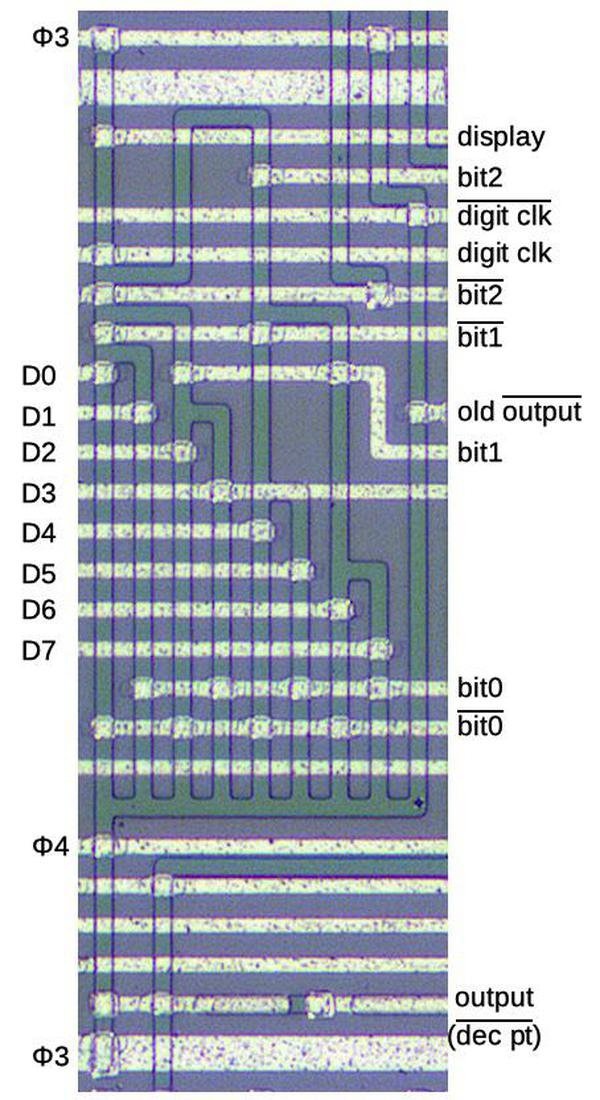A complex gate decodes the decimal point.

The circuit is implemented in four-phase logic as a single gate. The gate can be viewed as an 8-to-1 multiplexer that selects one of the eight digit (D) lines based on the bit value. This gate also includes a latch to hold the multiplexed value. Note that if the digit clock is 0, the AND gate at the bottom will cycle the output value (through an inverter, not shown), holding the value. When the digit clock is 1 (i.e. a digit has been read in), a new value from the multiplexer tree will be read. The branching tree structure is visible in the silicon structures above.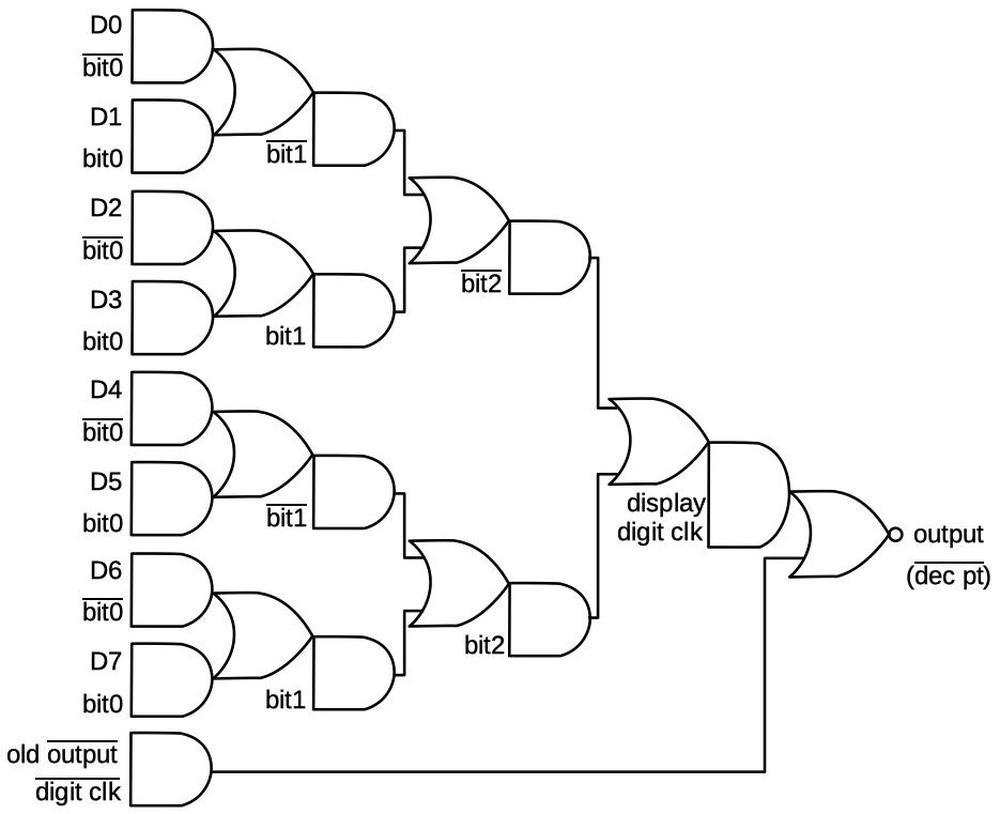Diagram showing the complex gate that decodes the decimal point.

### Other circuits

I won't describe the remainder of the circuits on the chip in detail. They were implemented using similar techniques, in particular shift registers. The keypress is converted to serial data with a latch and shift register, built from AND-NOR gates. The digit scan circuit is also a latch and shift register, with a gate to start a 1 value. This shift register is triggered by teh digit clock, so it shifts every 4 cycles. The circuit that divides the clock by 4 is a shift register to count four cycles.

## Conclusion

Although Sharp managed to fit the calculator circuitry onto five chips, it was soon overshadowed by single-chip calculators. In a few years, calculators shrank from the handheld but blocky Sharp EL-8 to credit-card-sized. The calculator market was highly profitable for a short time until the "calculator wars" caused calculator prices to drop from hundreds of dollars to a few dollars. Most of the hundreds of calculator manufacturers left the market, leaving Texas Instruments, Hewlett-Packard, Sharp, and Casio as the dominant manufacturers.

As for four-phase logic, its success peaked in the 1970s. Most notably, the company Four-Phase Systems created a 24-bit desktop computer in 1971 using four-phase logic, and Motorola bought the company in 1982. For the most part, though, microprocessors of the 1970s used static NMOS logic rather than four-phase logic. I haven't been able to find an explanation of why four-phase logic wasn't more widely used. My suspicion is that improvements in semiconductor technology in the early 1970s reduced the benefits of four-phase logic, specifically the introduction of depletion-load NMOS logic.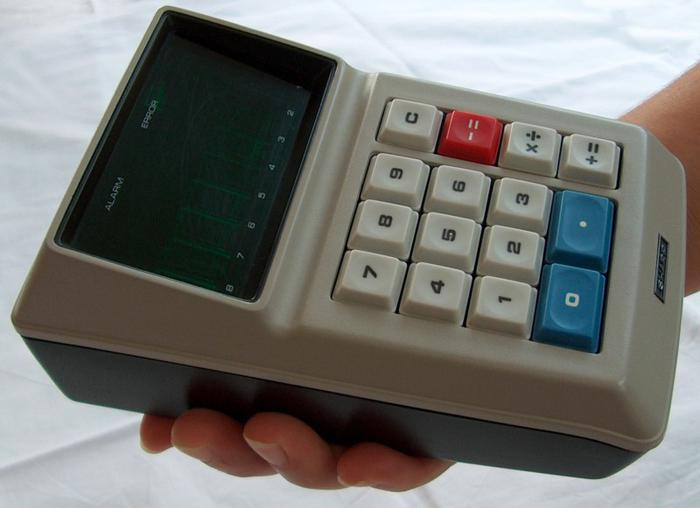The Sharp EL-8 calculator. Photo by Daniel Sancho (CC BY 2.0).

I plan to analyze the remaining three calculator chips so follow me on Twitter @kenshirriff for updates. I also have an RSS feed. Thanks to François Gueissaz for doing all the hard work of obtaining the calculator ICs, decapping them, and providing me with die photos and other information.

## Notes and references

1. The advantages of four-phase logic are discussed in a talk by Lee Boysel, an early proponent of MOS circuitry and four-phase logic. He founded the company Four-Phase Systems, which build a powerful desktop computer using four-phase logic. His interesting video on MOS history is here

2. The calculator display uses vacuum fluorescent display (VFD) tubes, developed as a lower-cost alternative to Nixie tubes to avoid paying patent royalties to Burroughs. Nixie tubes are similar to neon bulbs; there are 10 cathodes, each shaped like a digit, and applying 170 volts to a cathode causes the digit to light up with a neon glow.

The multi-segment VFD was invented in 1967 by Noritake Itron Corp. VFD tubes are vacuum tubes, sort of a cross between a triode and a low-voltage CRT. Unlike the "cold cathode" of Nixie tubes, the VFD's cathode is heated, causing electrons to boil off. These electrons are accelerated toward an anode by applying 25 volts to the anode, and cause a phosphor to light up when they hit the anode. A grid between the cathode and anode controls the electron flow; this is how a single tube is selected for multiplexing. The voltage in a VFD is much lower than a CRT, 25 volts instead of 25,000 volts. Another difference is that a CRT deflects the electron beam with deflection coils to create a pattern on the screen, while the VFD uses individual anodes that light up separately for each segment.

These Sharp calculators were the first calculators to use VFD tubes. The EL-8 calculator uses eight-segment Itron type DG10L tubes while the QT-8D calculator uses nine-segment DG10B tubes. The driver board has nine driver integrated circuits to interface between the calculator chips and the display tubes.

3. I'm skipping over a bunch of details of the calculator. For instance, some signals are active-high, while others are active-low, and some signals are shifted by half a clock. (The design is optimized to minimize the hardware, rather than being conceptually clean.) In this blog post, I'm describing the concepts of the circuitry rather than the cycle-exact details.

4. I haven't found many publications explaining four-phase logic. One is the article Four-phase logic is practical (1977). The 1969 master's thesis Basic design of MOSFET, four-phase, digital integrated circuits has a lot of information. The book MOS integrated circuits and their applications (1970) has a chapter on four-phase logic. See also Low-power VLSI implementation by NMOS 4-phase dynamic logic, published at the surprisingly late date of 2000.

5. Note that the gate is powered only by the clock; there are no power or ground connections. Although the four-phase gate are powered through the clock, the chip does have connections for power (-25V) and ground. Power and ground are used by the output pins so they can provide static signals with more substantial current. Ground is also used for the gate capacitors.

6. Most of the classic 1970's microprocessors used a two-phase clock. They used dynamic circuitry, typically for temporary data storage and timing, but the logic was typically static. The Intel 8086 used dynamic logic in a few places, such as the ALU, probably for performance reasons.

7. In most cases, four-phase circuitry alternates between φ1φ2 gates and φ3φ4 gates. A problem arises, however, if one path to a gate has an odd number of gates and another has an even number of gates. The solution is two more types of gates, one that precharges on phase 1 and samples on phase 3, and one that precharges on phase 3 and samples on phase 1. These gates are slower, but can interface between the earlier two types. Thus, four-phase logic has four types of gates, distinguished by the clock phases they use. Following the simple interconnection rules ensures that the circuit operates correctly.

The four types of four-phase gates are illustrated in A mathematical model characterizing four-phase MOS circuits for logic simulation. (1968) and Four-phase logic is practical (1977). (I'm pretty sure the second article has some errors in Figure 2 though.)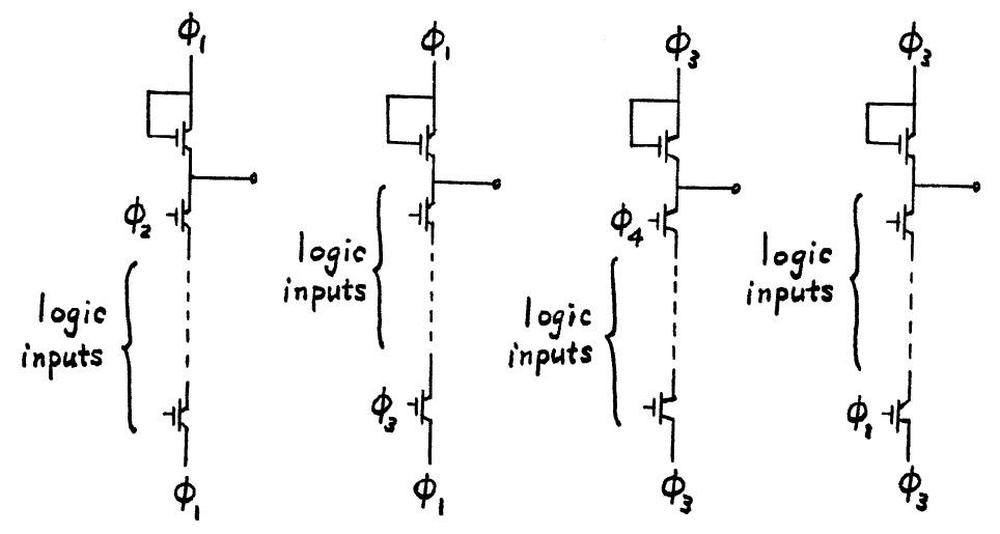The four types of four-phase gates.From A mathematical model characterizing four-phase MOS circuits for logic simulation.

Only certain combinations of four-phase gates can be connected. The diagram below shows that, for instance, the output from a type 1 gate can connect to the input of type 2 or type 3. A typical circuit alternates between Type 1 and Type 3. The calculator chip uses a few Type 2 gates and Type 4, for example when an extra inversion is required.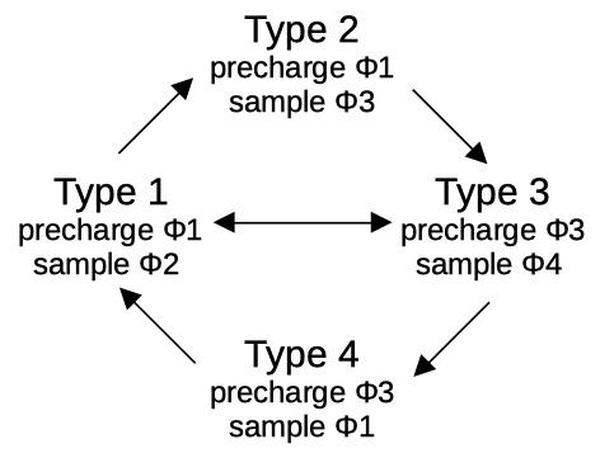Connections between four-phase gates must satisfy certain rules.

### Reverse-engineering the clock chip in the first MOS calculator

In 1969, Sharp introduced the first calculator built from high-density MOS chips, the QT-8D, followed by the handheld Sharp EL-8, the world's smallest calculator at the time.1 These calculators were high-end products, selling for \$345 (about \$1800 today). Integrated circuits at the time couldn't fit the entire calculator on one chip, so these calculators contained five ICs: an arithmetic chip, a decimal point chip, a keypad/display chip, a control chip, and a clock chip.

This blog post discusses the clock chip and how it generated the unusual four-phase clock signals required by the calculator. The die photo below, provided by calculator researcher Francois Gueissaz, shows the silicon die of the clock chip. the silicon substrate has a purple tint while the doped, conductive silicon is green. The metal layer on top is white. Around the edges, seven thin bond wires connect the die to the external pins.2 This chip has about 200 transistors and implements just a dozen moderately complex logic gates. While the density of this chip is absurdly low by modern standards, it illustrates the progress of MOS integrated circuits in the late 1960s.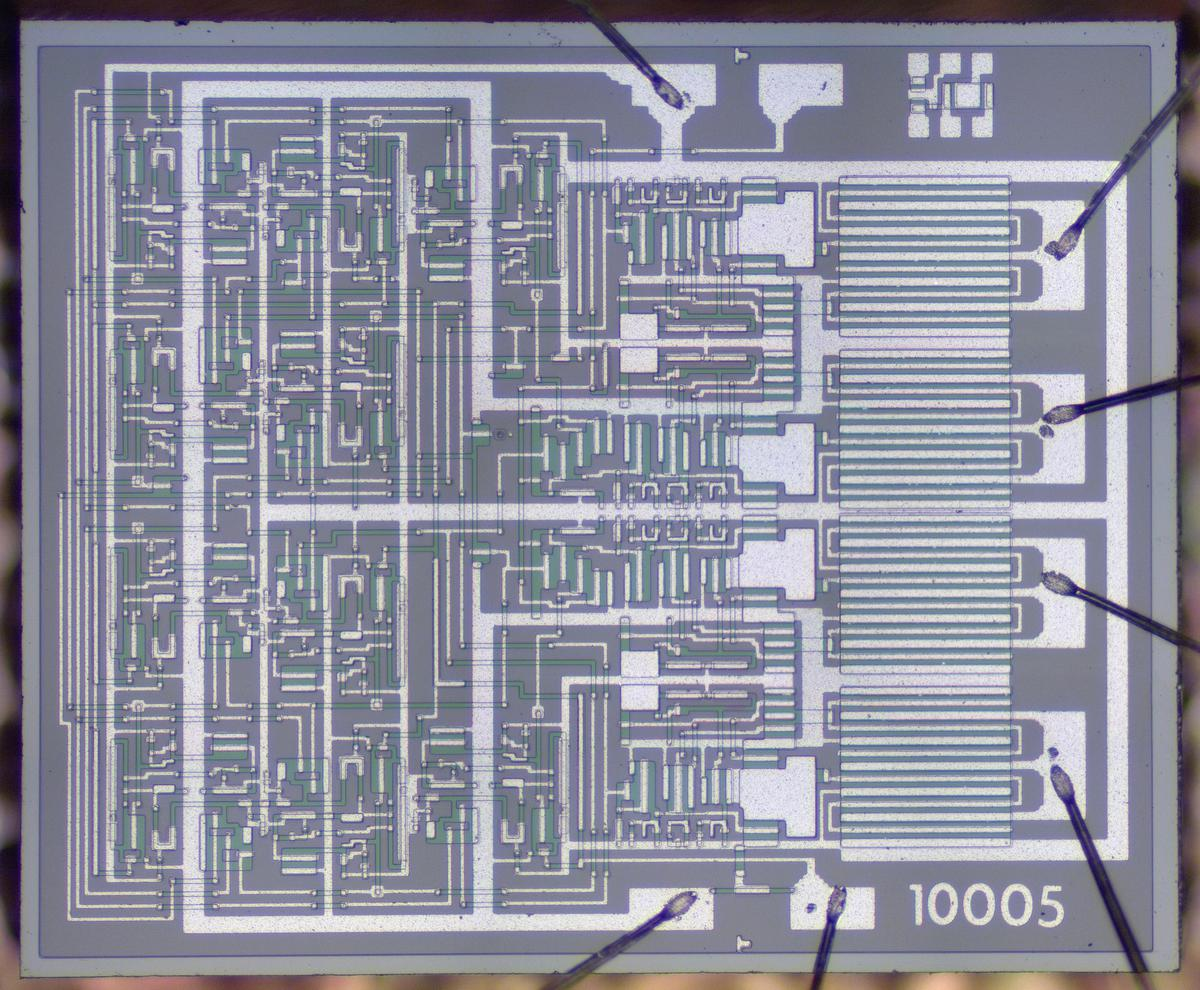Die photo of the CG2341 clock generator. This photo (and many others) courtesy of Francois Gueissaz.

Although computers now all use MOS integrated circuits, the path to MOS was rocky, with MOS integrated circuits viewed as slow and unreliable in the 1960s.4 Handheld calculators were a good match for the characteristics of MOS, though: they needed to be compact and lightweight with low power consumption, but computational speed was not important. In 1969, the Japanese calculator company Sharp signed a \$30 million deal with Rockwell for this MOS-based calculator chipset, the largest MOS order in history at the time. The five chips were implemented by the Autonetics division of Rockwell.3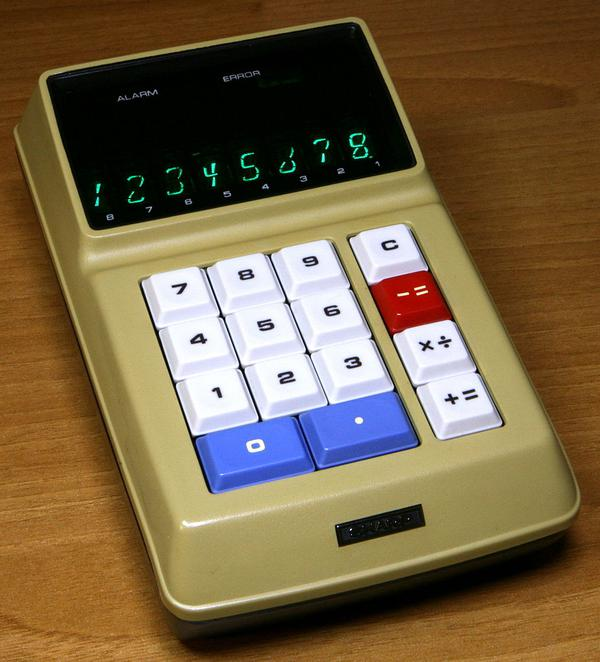The Sharp EL-8 calculator. Note the unusual 8-segment display for the digits. Photo by Mister rf (CC BY-SA 4.0).

Although the Sharp calculator (above) was handheld, you can see that it was rather thick and chunky, with unusual 8-segment vacuum fluorescent display tubes for its display. The photo below shows the circuit board inside the calculator. The board is dominated by the four large integrated circuits with circular golden lids. These integrated circuits were packaged as 42-pin ceramic ICs with staggered pins. Unlike modern printed circuit boards, the traces on this board are curved, showing its hand-drawn layout.The circuit board for the Sharp EL-8 calculator. The clock IC is the small metal-can package in the middle. Photo from Mister rf (CC BY-SA 4.0).

The clock IC is packaged in the small 10-pin metal can, marked with a blurry Rockwell logo (the inset shows the logo). This part number is CG1121 (probably standing for Clock Generator) and is similar to the CG2341 I examined. The date code 7047 indicates this IC was manufactured in the 47th week of 1970, i.e. late November.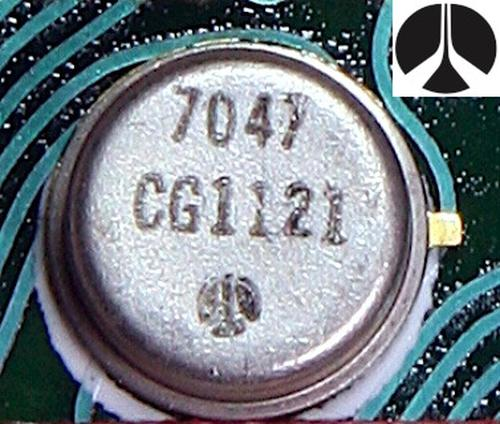The clock integrated circuit was packaged in a 10-pin metal can. The logo on the integrated circuits isn't clear, but it is the Rockwell logo as shown in the inset.

Cutting the top off the metal can integrated circuit reveals the tiny silicon die. Although the metal can has 10 pins, only seven pins are wired to the die. The metal tab at the top of the photo indicates pin 1 of the integrated circuit.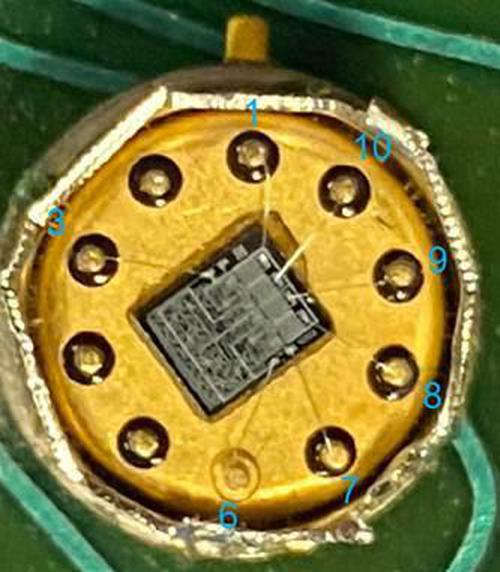The metal can of the CG2341 with the lid removed, showing the silicon die inside.

Why do the calculator chips require a complex four-phase clock? In 1966, Autonetics invented a technique for building logic circuits called four-phase logic. Unlike standard static logic gates, these logic gates held values dynamically using the capacitance of the wiring. The four-phase clock stepped the gates through sequences of precharging and then computing the logic function. This sounds complicated, but four-phase logic had ten times the density of standard logic gates, as well as using 1/10 the power and having 10 times the speed. As a result, many early high-density MOS chips used four-phase logic.5

## Constructing transistors, resistors, and capacitors

Transistors are the key component of the chip. The diagram below shows a metal-gate PMOS transistor, the (somewhat primitive) type of transistor used in this IC. At the bottom, two regions of silicon (green) are doped to make them conductive, forming the source and drain of the transistor The gate is formed by a metal strip between the silicon regions, separated from the silicon by a thin layer of insulating oxide. (These layers—Metal, Oxide, Semiconductor—--give the MOS transistor its name.) The transistor can be considered a switch between the source and drain, controlled by the gate. To simplify the behavior, a PMOS transistor turns on when the gate is pulled negative (-25 volts), while the transistor turns off when the gate is at 0 volts. (These early PMOS transistors required an inconveniently large negative voltage.)Structure of a PMOS metal-gate transistor.

The photos below show transistors on the die as they appear under a microscope. The silicon and metal layers match the diagram above; the doped silicon is greenish while the metal layer on top is white. The gate is formed where the metal and silicon overlap, with a faint oval where the oxide is thinned. These transistors are three different sizes: the wider transistors allow higher current. The transistors are carefully sized in the circuits based on the required current.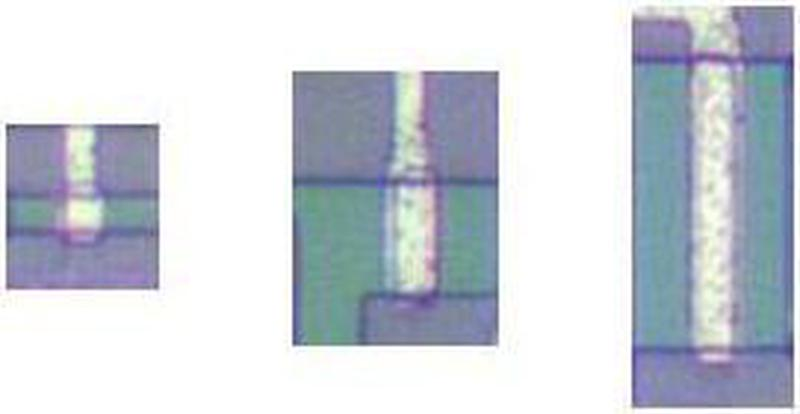Three transistors of various sizes, as seen on the die.

The next important component is the resistor; the photo below shows three resistors. These resistors may look like transistors, and that's because they are transistors. While the transistors above were widened to support more current, these transistors are made longer so the long path reduces the current flow through the transistors. This makes them act as resistors. The metal gate of these transistors is tied to -25 volts, so the transistors are always on, rather than operating as switches.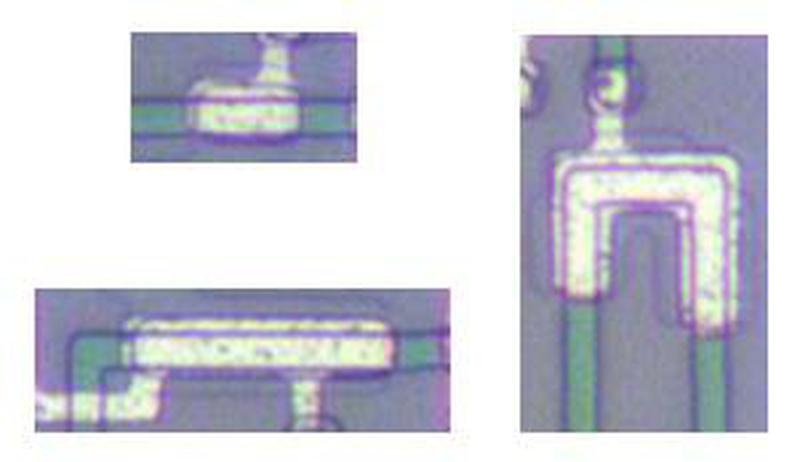Resistors of various sizes.

The final important component of the integrated circuit is the capacitor. A capacitor is formed by using metal for one plate and doped silicon (green) for the other plate, separated by the insulating oxide layer. The photo below shows two small capacitors and one large capacitor, at the same scale. The large capacitor is used in the output circuitry; the metal stripes above and below it are transistors that drive it.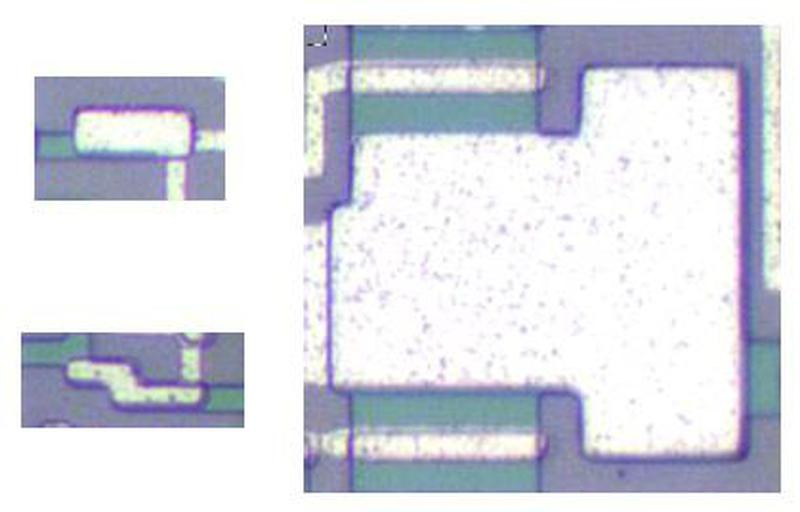Two small capacitors and one very large capacitor.

## Implementing an inverter and NAND gate

With these components, logic gates can be constructed. The schematic below shows how an inverter is implemented in the IC. The layout of the schematic matches the die image underneath, so hopefully the transistors and capacitor can be recognized. If the input is low, the input transistor turns on, pulling the output to ground (i.e. high). If the input is high, the input transistor turns off and the "bootstrap load", the tricky circuit on the right pulls the output to -25V (i.e. low). Thus, the circuit inverts the input.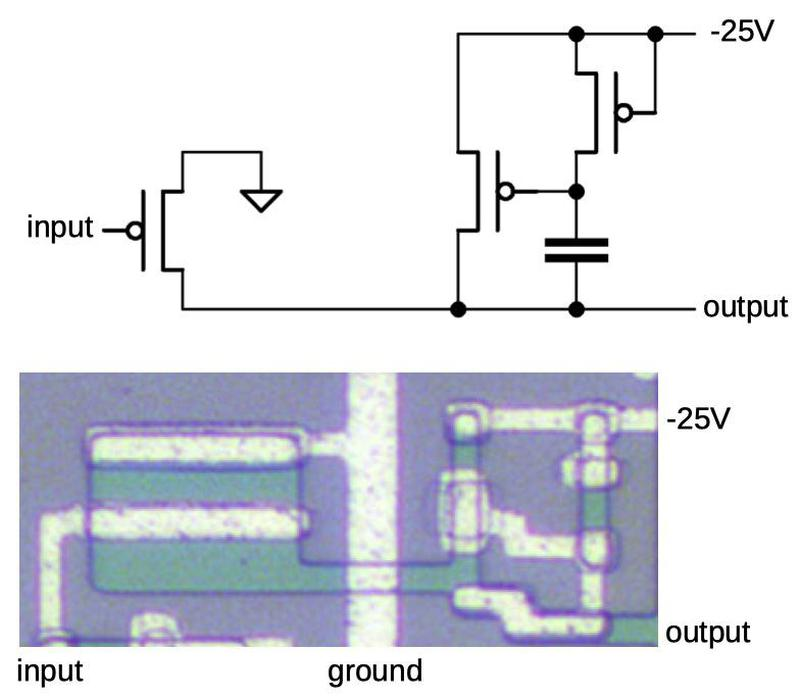An inverter using a bootstrap load.

Conceptually, you can think of the bootstrap load as a pull-down resistor. The implementation is complex to compensate for the poor characteristics of transistors at the time. The capacitor acts as a charge pump, providing a necessary voltage boost when the circuit switches. (For more details on bootstrap loads, see my earlier article.)

The implementation of a NAND gate is similar to the inverter above, but with multiple input transistors in parallel. If any input is low, the corresponding input transistor turns on, pulling the output to ground (i.e. high), as required by a NAND gate.

## The NAND delay gate

The die photo below shows the functional blocks of the clock chip. Eight NAND gates (red) form an oscillating 4-bit shift register. Four gates (yellow) generate the four-phase clock signals from the shift register outputs. Finally, four output driver circuits (orange) amplify these signals to produce high-current outputs.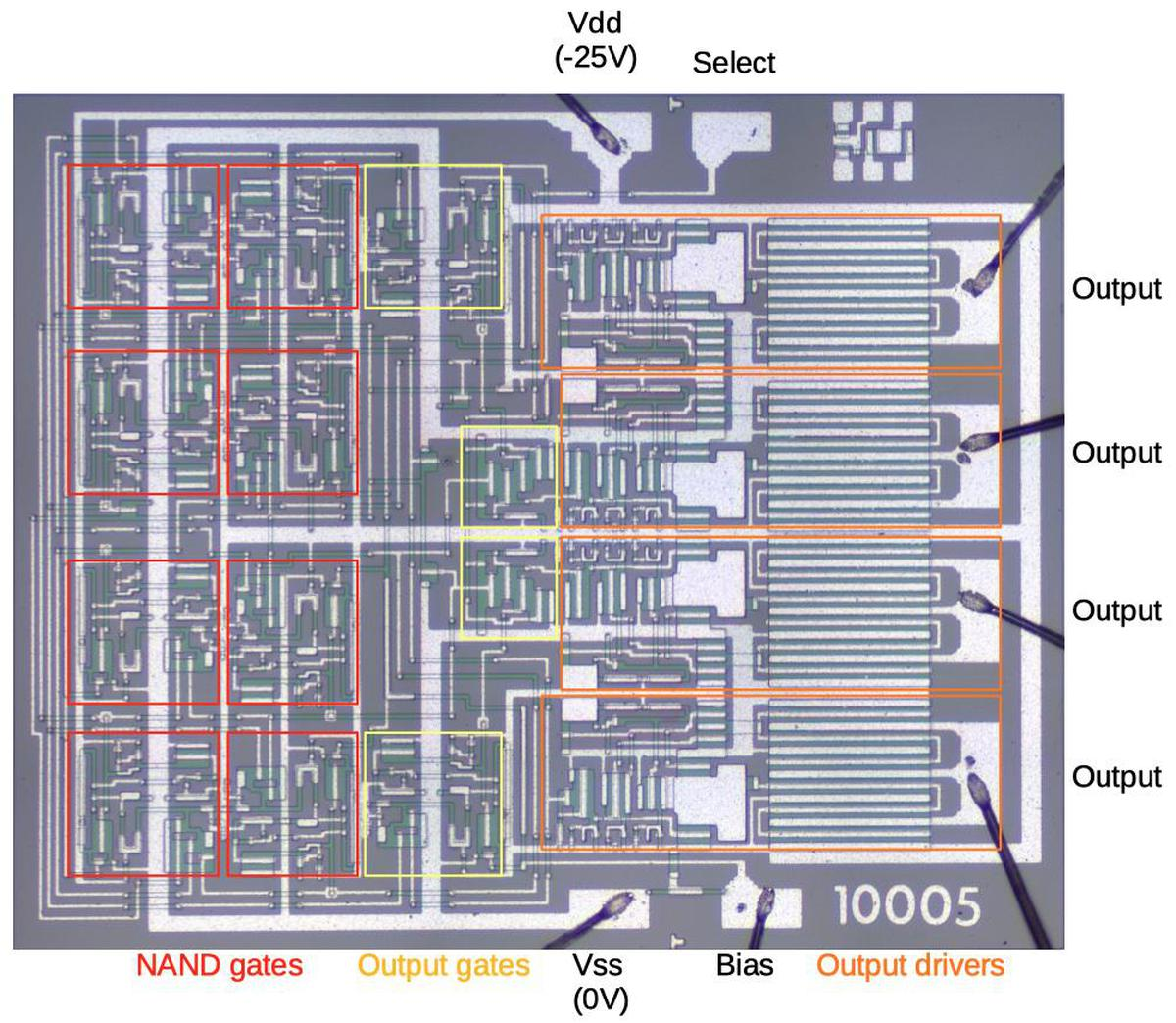The clock chip die with key components labeled.

The main building block of the clock chip is a NAND gate that has a delay when its output goes low. This delay creates the timing of the clock signal.6 The diagram below shows how the gate is constructed; the schematic corresponds to the layout of the circuit on the die. The delay makes this circuit somewhat complex and partially analog, but I'll try to explain it.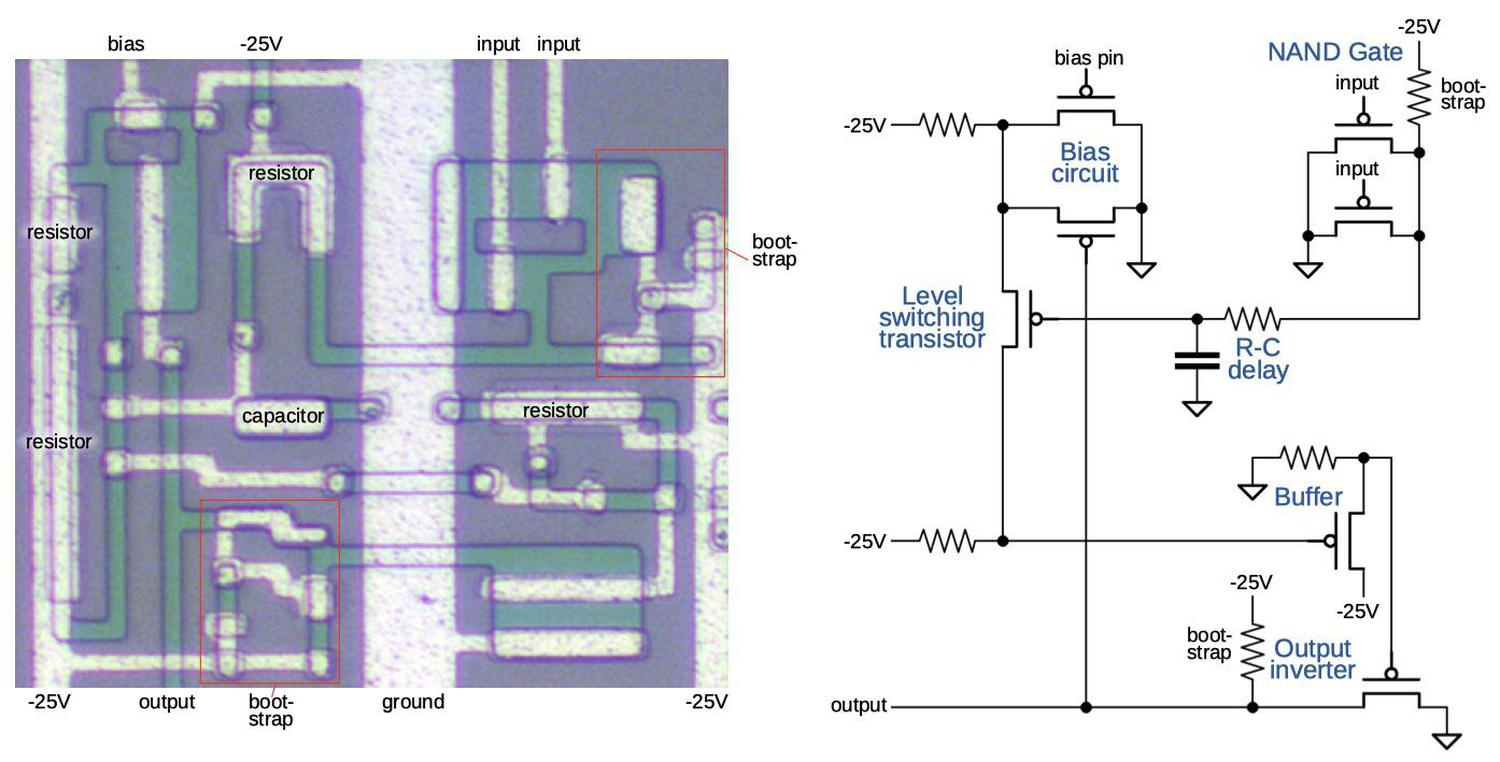The NAND delay gate uses an R-C circuit to provide the delay. For simplicity, the bootstrap load is represented by a resistor.

The NAND circuit is in the upper right; two input transistors and a bootstrap load implement the NAND circuit described earlier. The output of the NAND gate goes through a resistor-capacitor circuit. This delays the output as the capacitor slowly charges through the resistor. The speed of the clock is controlled by the bias pin, which sets a threshold voltage. This voltage controls the point in the resistor-capacitor curve when the level switching transistor turns on.7 By lowering the voltage on the bias pin, the transistor switches sooner, increasing the clock speed. The typical clock speed is 60 kHz, a slow clock even compared to early microprocessors, but calculators didn't require much speed.

When the level switching transistor turns on, it pulls the buffer high,8 and driving the inverter's output low. The inverter has a bootstrap load to provide sufficient output current. Finally, the output is fed back to the bias circuit, probably to sharpen the transition and provide hysteresis. To summarize, this complex circuit implements a delayed NAND gate. It is the key functional block of the chip, repeated ten times.

## The clock shift register

The clock is built from a 4-stage shift register. The idea is that each stage of the shift register shifts its bit to the right, after a delay. The bit on the right is inverted and shifted into the left side of the shift register. Thus, the shift register implements a ring counter, first shifting in 1's at the left and then shifting in 0's: the bit pattern is 0000, 1000, 1100, 1110, 1111, 0111, 0011, 0001, and back to 0000. This complete cycle corresponds to one 60 kilohertz clock cycle for the calculator.

The schematic below shows how the shift register is built from eight cross-coupled NAND gates with delay, using the circuit described earlier. Each pair of NAND gates forms a latch, storing either a 0 or a 1. The latch outputs are labeled Q0 through Q3 while the inverted outputs are labeled Q0 through Q3. The outputs from each latch are connected to the inputs of the next stage, so the bits are shifted to the right. Note that the wires from the last stage back to the first stage are crossed; this causes the bit to be inverted. Each stage consists of two cross-coupled NAND gates, forming a latch that holds one bit. If the delay is decreased (through the bias pin), the speed of the shift register increases, increasing the clock speed.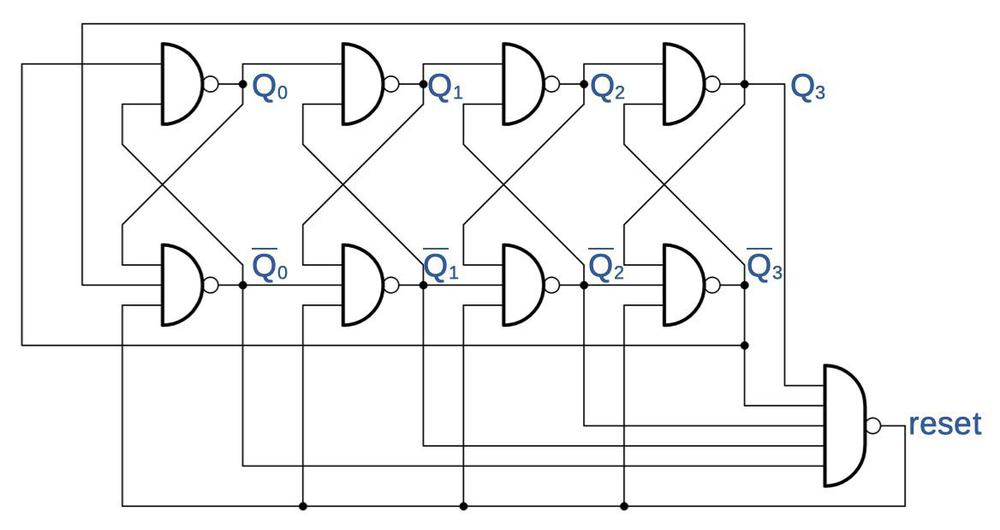The 4-stage shift register.

The shift register must be initialized to the proper state, which is the job of the reset gate. When the shift register is powered up, the reset gate initializes the latches to hold zeros by pulling the lower inputs to the latches low.

## Output circuit

The output circuitry generates the four clock phase outputs from the shift register values. Two phases come from the last shift register stage and its complement. The other two phases are more complex. An unwired "select" pin selects between two outputs for these pins; presumably this pin was wired in other versions of the clock chip to provide different clock signals for a different calculator. In the normal case, these clock outputs are formed by NANDing together two shift register outputs to produce a shorter pulse.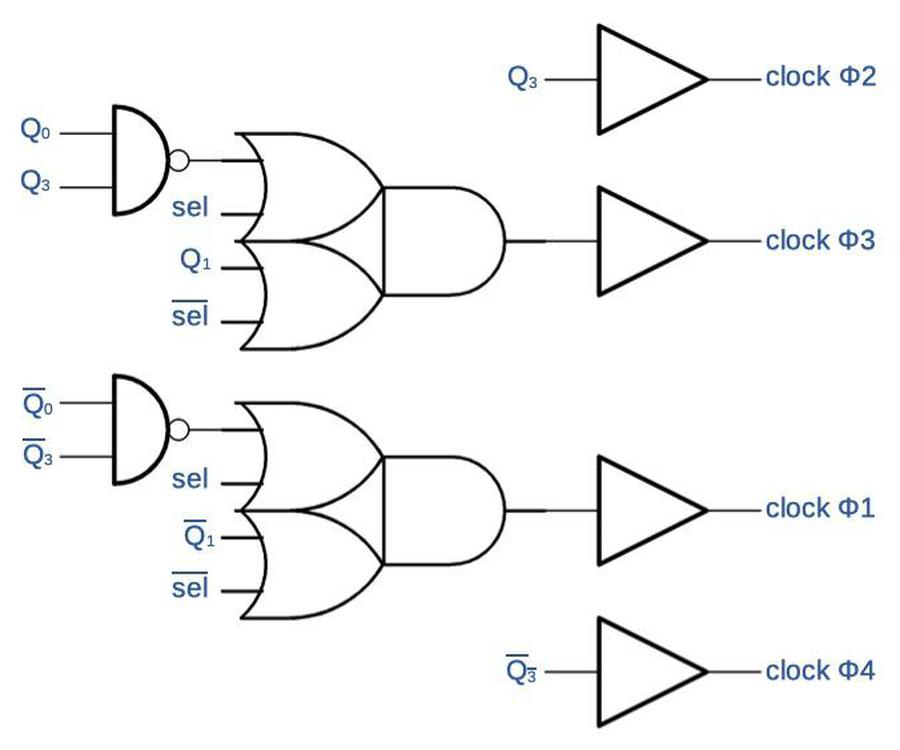The output circuit produces four clock outputs from the shift register values.

The photo below shows one of the output buffers. The output signal enters at the left, travels through the buffer circuitry, and exits the chip through the bond wire on the right. The right half consists of two large transistors to provide the high output currents: one transistor pulls the output up to ground, while the other transistor pulls the output down to -25V. The remainder of the circuitry amplifies the small internal signal so it can drive the output transistors. Note the large bootstrap capacitor near the center; it helps drive one of the output transistors. There are also much smaller bootstrap capacitors in the upper left. This output buffer circuit is repeated four times, once for each output pin.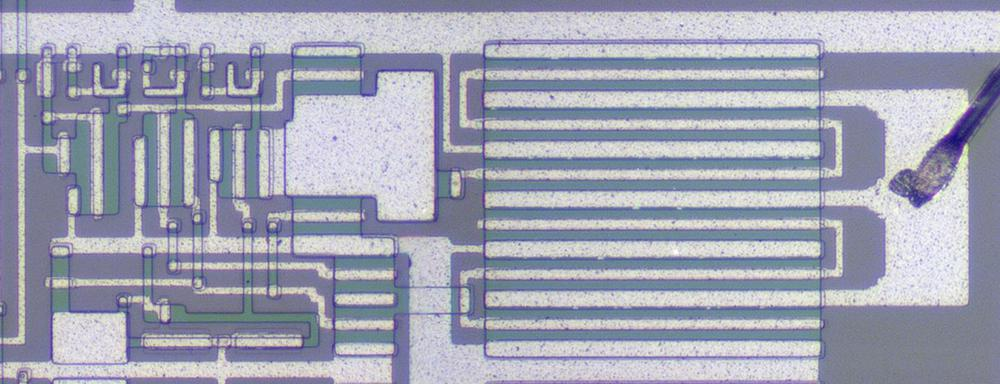One output buffer as it appears on the die.

The output buffer transistors must be large due to an unusual characteristic of four-phase logic. Normal clocked logic uses the clock signals for timing, while the logic gates are connected to power and ground. In four-phase logic, however, the clock signals provide the power for the logic gates; there are no separate power and ground connections. When the gates are precharged and discharged by the clock signals, this provides the power for the gates. Thus, four-phase logic requires relatively high-current clock signals, since they are powering the circuits.9

To see the chip in action, the oscilloscope trace below shows the four clock outputs as measured from the chip. The yellow and blue traces are the main phases; note that the active (low) parts do not overlap. The magenta and green outputs are active during the first part of the yellow and blue phases, respectively. These clocks are used to precharge the logic circuits. (The clock phases match those on Wikipedia's four-phase article, except the polarity is reversed because of the PMOS transistors.)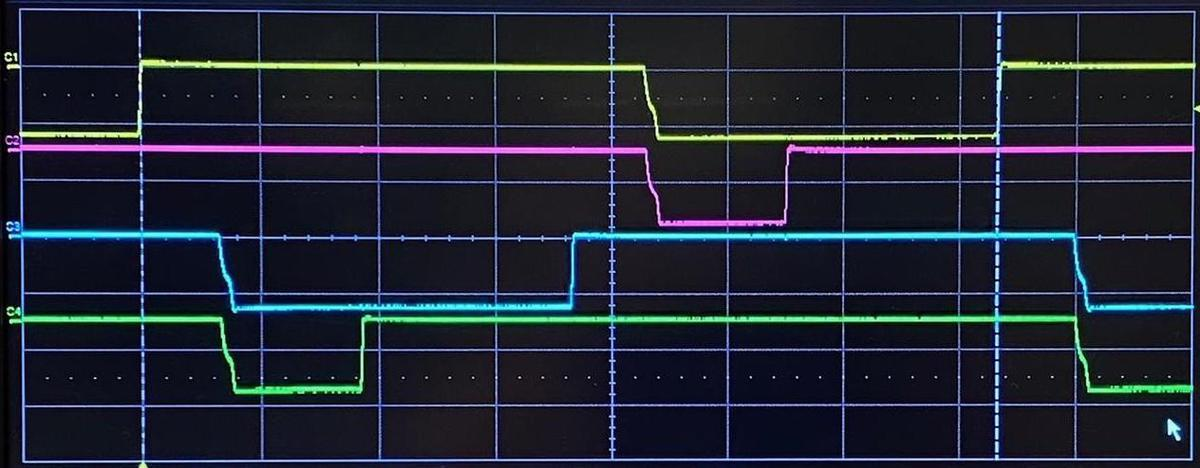Oscilloscope trace showing the four output phases from the clock chip.

## Conclusion

Rockwell fit a calculator onto five chips, making the handheld calculator possible. However, Texas Instruments, Mostek, and other companies soon fit all the circuitry onto a single chip, creating the calculator-on-a-chip. Selling calculators was highly profitable for a short time and 11 million calculators were sold in the US in 1974. Although calculators sold for hundreds of dollars in 1969, competition and the improvements in technology caused calculator prices to plummet to \$15 by 1975. The profit margin collapsed during the "calculator wars"; Texas Instruments alone lost \$16 million in 1975.4

Although the calculator market was risky, the massive sales of calculators provided an important boost to MOS chip technology in the early 1970s, and thus the computer industry. In particular, microprocessors started with the Intel 4004, a chip designed for a calculator. And microcontrollers were created out of Texas Instruments' line of calculator chips. While a chip such as the CG2341 clock generator is trivial by modern standards with about 200 transistors, it provides a historical window into how chips were constructed in the early days of MOS ICs.

Thanks to Francois Gueissaz for doing all the hard work of obtaining the calculator ICs, decapping them, and providing me with die photos and other information. I announce my latest blog posts on Twitter, so follow me at kenshirriff. I also have an RSS feed.

## Notes and references

1. See this interesting vintage commercial for the Sharp EL-8 calculator for more information.

2. Measuring the die photo, I believe this chip uses a 15 µm process, so the transistors and features are very large by modern standards. (This is why five chips were required to implement the calculator.) In comparison, many modern chips use a 14 nm process, so the width of a modern transistor is roughly 1000 times smaller, and the area is roughly a million times smaller. This shows the amazing progress in silicon technology described by Moore's Law.

3. It's hard to follow the spin-offs and acquisitions of the companies involved. Autonetics was founded as the research laboratory for North American Aviation in 1945. Among other things, Autonetics developed guidance computers for the Minuteman missile. Although North American Aviation is mostly forgotten now, it was a major aerospace company, building everything from the P-51 Mustang in World War II to the command and service module for the Apollo landing. It merged with Rockwell in 1967, becoming North American Rockwell. In 1970, about 800 employees from Autonetics were split off to form North American Rockwell MicroElectronics to develop and manufacture commercial integrated circuits. This later became Rockwell Semiconductor, then spun off into Conexant, which was later acquired by Synaptics. Rockwell was sold to Boeing in 1996.

Sharp, on the other hand, started as Hayakawa Metal Works in 1924, eventually being renamed Sharp Corporation in 1970. (The name came from the Ever-Sharp mechanical pencil, one of Hayakawa's early inventions.) Foxconn bought the majority of Sharp in 2016; Foxconn, also known as Hon Hai Precision Industry, is a Taiwanese electronics manufacturer. Although best known for manufacturing the iPhone for Apple, Foxconn is estimated to manufacture 40% of the world's consumer electronics.

4. Much of the historical information in this post comes from the books To the Digital Age and History of Semiconductor Engineering. These books provide a detailed look at the rise of MOS integrated circuits.

5. One of the main proponents of four-phase logic was Lee Boysel, who founded a company Four-Phase Systems around it. The company built 24-bit computers, which were some of the earliest MOS-based computers. Boysel's EECS presentation describes the advantages of four-phase logic.

6. One important characteristic of the delayed NAND gate is that the delay is much larger when the output goes low than when the output goes high. This ensures that the output clock phases do not overlap while active (low). This is necessary for four-phase logic to ensure that logic gates don't conflict with each other.

7. The level switching transistor (like other PMOS transistors) will turn on when the gate voltage is lower than the source voltage by Vt (the transistor's threshold voltage). Thus, by controlling the bias voltage on the transistor's source, the transistor can be made to turn on sooner or later, controlling the frequency.

8. Note that the buffer circuit is constructed "backward" compared to a standard PMOS inverter. A PMOS inverter has the transistor connected to ground with a load resistor to -25V, while the buffer has the transistor connected to -25V and the load resistor to ground. I think it is constructed this way to shift the voltage levels from the level switching transistor.

9. Although the four-phase clocks power the logic gates, the chips also have regular power and ground connections. These power the output pins since the current demands are too large to be reasonably satisfied by the clocks.